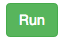# 1.2. Compute with Numbers¶

Computers were first used for numeric calculations. You may be used to doing calculations with a calculator, but calculations are often easier if you can name the numbers you are working with. When you name a number, or the result of a calculation, you are creating a variable. A variable is a name associated with computer memory that can hold a value and that value can change or vary. One example of a variable is the score in a game. The score starts off at 0 and increases as you play the game.Both the score and high score in the game Angry Birds would be represented by a variable.¶

One thing that you might want to calculate is the monthly payment on a loan for a car. To do so, you can use this mathematical formula:

$$A = P \frac{r(1 + r)^n}{(1 + r)^n - 1}$$

That formula uses the following variables:

• P : The principle - the amount of the original loan

• rThe monthly interest rate expressed as a decimal value. Loans are often described in

terms of an APR or Annual Percentage Rate that must be divided by 1200 to produce the right value for r.

• n : The number of monthly payments

It calculates the value A which is the payment amount.

The Python program below sets some values for these variables and then does the calculations needed to produce the payment.

Press thebutton below to make the computer execute these steps. The output from this program will be displayed to the right of the program.

You can only use the Save and Load buttons if you are logged in. The Save button will save the current program and the Load button will load a saved program.

Currently the code is calculating the payment for a $10,000 loan at an APR of 4.9% over 60 months. Try changing the numeric values for P, APR, and n in the program above, and press the Run button to calculate the payment for a different loan. Note that some of the symbols used in Python are different than those we normally use for math: * means “multiply” and ** means “to the power of”. Also visible in the code are some comments. Comments are pieces of text that come after a # symbol, like #$10,000 - notice we don't use ,'s in numbers. Python will ignore these comments and they are colored differently than the code to indicate that they are not actual code. Comments are used by programmers to leave notes to themselves and others about the code. Try deleting a # in the program above and then running it. You will get an error message because now Python is trying to run the note as if it was code.

Note

Notice how naming the values (using variables) for things like n makes it easier to see which values to change to make the program calculate a different loan.